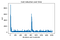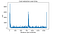In my earlier post, we have discussed about what is Gradient Descent, how it works and Importance of Learning Rate. Also, we discussed how to make sure that gradient descent is working properly.

To recall what was gradient descent, let us define it once again. Gradient Descent is one of the most popular optimization algorithms for finding optimal parameters for the model. Our goal is to find the parameter which minimize the cost function.

Here, we will talk about different types of Gradient Descents defined on the basis of how we use the data to calculate the derivative of the cost function in gradient descent. Depending upon the amount of data used, the time complexity and accuracy of the algorithms differ with each other.

In this type of Gradient Descent, the whole training data set is used to compute the gradient of the cost function. It means that whole training data set is checked upon for any errors and then it is updated back. This whole process is called a cycle and a training epoch. Since, the data set it can be called as a batch, hence, it is called as Batch Gradient Descent.

# Pros

• During training, we may use a fixed learning rate without thinking of its decay.
• It is a deterministic algorithm.
• It is computationally efficient since it produces a stable error gradient and a stable convergence.
• It guarantees to converge to global minima in case of convex error surfaces and to local minima in case of non-convex surfaces.

# Cons

• Since it uses the whole data set at every iteration, hence, it makes the computation very, very slow especially when the data set is very large.
• When we go through all the examples present, then learning happens at every step and it may be possible that some examples are redundant and do not contribute much to update. Hence, checking them over and over again is time-consuming and useless.
• It requires that the whole training data set is in memory and also available to the algorithm.
• We can get the local minimum by this approach, but it is not necessary that the local minimum is also the global minimum.

One of the common ways to avoid the trap of going to a local minimum is modifying the weights after each processed input of the training set. When all inputs from a training set are processed, one epoch is done. For getting best results, multiple epochs should be done. This explained process is called — Stochastic Gradient Descent. Also, by doing so, we are minimizing the possibility of another problem arising — over-fitting.

## What is over-fitting?

Over-fitting is a situation in which neural networks perform well on the training set, but when we give them the real values, they don’t perform well. This happens since they are trained to solve the specific problems mentioned in the training set and are unaware of the real ones they get later.

In this Gradient Descent, each example is updated one by one. This makes Stochastic Gradient Descent much faster than the Batch Gradient Descent as it has to manipulate less amount of data at each iteration.

# Pros

• Frequent updates improve the model.
• The frequency can also result in noisy gradients which may cause the error to increase instead of decreasing it.
• Allows the use of large data sets as it has to update only one example at a time, hence fewer instances are to be recognized by the model at a time.

# Cons

• The frequent updates are more computationally expensive.
• Due to its stochastic (random) approach, this algorithm is less regular than the previous one.
• This algorithm may also result in a local minimum but not in the global minimum.
• Variance is very large as the objective function fluctuates heavily on one example at each learning step.Cost function with respect to the number of iteration, Source: 

This is the best method. This type of Gradient Descent is a combination of the above two Gradient Descents. In the above two Gradient Descents, we used either full data set or a single instance to compute the gradient. In this, we will use mini batches of instances to update the model.

This algorithm reduces the noise occurred in the Stochastic Gradient Descent and still is more efficient than Batch Gradient Descent. It splits the data set in batches according to our choice generally in 50 to 256 examples, chosen at random.

# Pros

• It is less erratic.
• It gets closer to minimum.
• It leads to more stable convergence.Cost Function with respect to the number of iterations, Source: 

Below is a graph that shows the gradient descent’s variants and their direction towards the minimum:

# Conclusion

In this blog, we discussed about the three variants of Gradient descents. We discovered that if any gradient descent is to be used in Deep Neural Networks then Mini Batch Gradient Descent is recommended as it is the best.

In our next blog, we will look for Convolutional Neural Network.

Will meet you soon in the next blog. Leave comments for any suggestions or query.

Written by

Written by

## Dr. Sunil kumar Jangir

#### B.E.(Hons.) | M.Tech(Gold Medalist) | Ph.D | Department of Information Technology JECRC, Jaipur

Welcome to a place where words matter. On Medium, smart voices and original ideas take center stage - with no ads in sight. Watch
Follow all the topics you care about, and we’ll deliver the best stories for you to your homepage and inbox. Explore
Get unlimited access to the best stories on Medium — and support writers while you’re at it. Just \$5/month. Upgrade